# Exploring the Properties and Applications of Triangles: A Comprehensive Guide

Triangles are one of the most basic and fundamental shapes in geometry, with three sides and three angles. Despite their simplicity, they have significant applications in various fields such as mathematics, engineering, architecture, and art. Understanding the properties and classifications of triangles is essential in many practical scenarios, including calculating the angles of a building's roof, designing a bridge, or even creating artwork. In this comprehensive guide, we will explore the different properties and classifications of triangles, and delve into their various applications in our daily lives.

# Exploring the Properties and Applications of Triangles: A Comprehensive Guide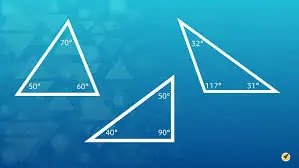This guide aims to provide a thorough understanding of the various properties and applications of triangles. We will begin by discussing the basic properties of triangles, including the relationship between their sides and angles, and the Angle Sum Theorem. We will then explore the different types of triangles based on their sides and angles, including equilateral, isosceles, scalene, acute, obtuse, and right triangles. Additionally, we will delve into the various applications of triangles in different fields of life.

## Properties of Triangles

A triangle is defined by its three sides and three angles. The sum of the three angles of a triangle is always 180 degrees. This is known as the Angle Sum Theorem. If we denote the angles of a triangle as A, B, and C, then we can write this theorem as:

A + B + C = 180 degrees

The sides of a triangle are denoted by a, b, and c, and the opposite angles are denoted by A, B, and C, respectively. The side opposite to angle A is denoted by a, and so on. The length of each side and the measure of each angle can be calculated using trigonometry.

## Classification of Triangles

A triangle has overall six elements (03 sides + 03 angles) therefore they have 06 types based on their sides and angles.

### Based on Sides:

The following are 03 types of triangles based on their sides.

Equilateral Triangle: A triangle in which all sides are equal is called an equilateral triangle. The measure of each angle in an equilateral triangle is 60 degrees.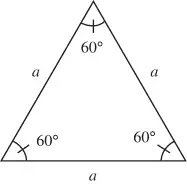Equilateral Triangle

Isosceles Triangle: A triangle in which two sides are equal is called an isosceles triangle. The measure of the angles opposite to the equal sides is also equal.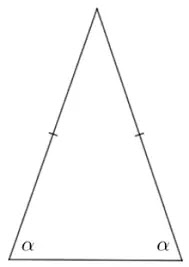Isosceles Triangle

Scalene Triangle: A triangle in which all sides are different is called a scalene triangle. The measure of each angle in a scalene triangle is different.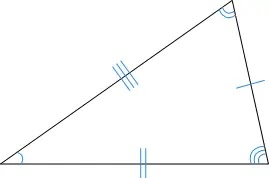Scalene Triangle

### Based on Angles:

The following are 03 types of triangles based on their angles.

Acute Triangle: A triangle in which all angles are acute (less than 90 degrees) is called an acute triangle.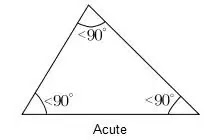Acute Triangle

Obtuse Triangle: A triangle in which one angle is obtuse (greater than 90 degrees) is called an obtuse triangle.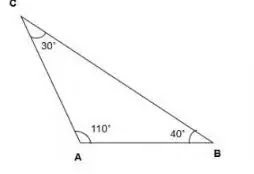Obtuse Triangle

Right Triangle: A triangle in which one angle is a right angle (90 degrees) is called a right triangle.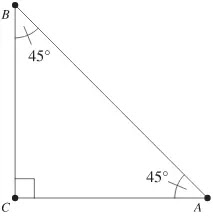Right Triangle

## Applications of Triangles

Triangles have important applications in many fields, some of which are:

Trigonometry: Trigonometry is the branch of mathematics that deals with the relationships between the sides and angles of triangles. Trigonometric functions such as sine, cosine, and tangent are used to calculate the unknown sides and angles of triangles.

Engineering: Triangles are used in engineering to calculate the stress and strain in structures. The Pythagorean theorem, which states that the square of the hypotenuse of a right triangle is equal to the sum of the squares of the other two sides, is used to calculate the distance between two points in 3D space.

Architecture: Triangles are used in architecture to create stable structures that can withstand external forces. Triangular shapes are often used in roof designs to distribute the weight of the roof evenly and to prevent sagging.

Art: Triangles are used in art to create balance, harmony, and tension. Triangular shapes can be used to create perspective and depth in paintings and drawings.

## Conclusion

Triangles are simple yet important shapes in geometry. They have many applications in various fields and are essential in understanding trigonometry and calculating unknown sides and angles. The classification of triangles based on sides and angles helps to identify their properties and use them in specific situations. Triangles are not just mathematical concepts, but they are also used in everyday life and art, making them fascinating subjects to explore.